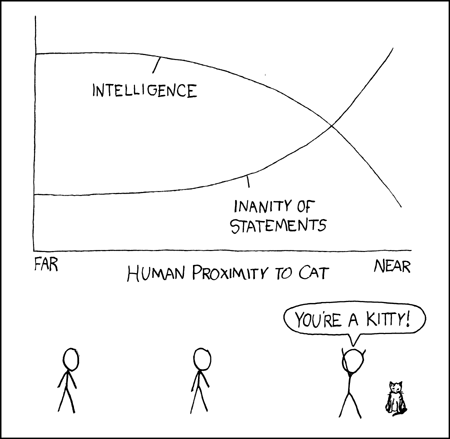# March, 2015Archive

Mar 23

## Cat Proximity and its effect on the human intelect

Posted in Comic Strips,

Randall Munroe drew a lovely comic strip graphing the effects of proximity to a cat on the human intellect:(You can view it here: http://xkcd.com/231/).

In differential calculus I asked students to describe the graphs using their knowledge of calculus.  Statements about both the first and second derivative are expected.

In Intermediate Algebra here at UDM we study qualitative graphs, so I also asked my Algebra students to describe the graph.  These students have to say things like the intelligence of the human is decreasing, but at an ever faster rate.

One caution with this comic: some students do not know what “inanity” means, so I use this problem on the review sheet rather than the exam.

Mar 18

## Roots, factorials, trig, and summation notation

Posted in Comic Strips,

In this FoxTrot strip Paige has been cramming for her math final and thus is answering all questions in mathematical notation.

http://assets.amuniversal.com/b07d90305e30012ee3bf00163e41dd5b

(Its the May 22, 2005 strip, if the link doesn’t work.)

In precalculus I asked the students:

1) What temperature will it be tomorrow?  Is it going to be hot tomorrow?

(The answer is sin^{-1}(1) =90 degrees, so it will be hot).

2) What does the girl (Paige) want for snack?

(The answer is 3.14159265359…, so she wants pie).

In calculus of sequences and series, you could also ask what the discount was in the fourth panel, which requires computing the sum of an infinite geometric series.

In an algebra class one could ask what time Paige went to bed (\sqrt{121}= 11:00) or What is on TV? (4!=24).

Mar 16